professorclark.net      ACT/SAT PRACTICE

Algebra IHow to Study MathGeometryACT/SAT Test Practice Algebra II Becoming a Pro AthleteAnalysisTeacher ResourcesCalculators, Dictionaries, other Online ToolsHayti High
Hayti, MO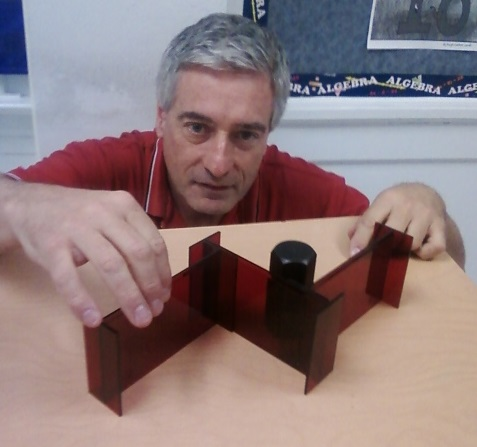Go Indians!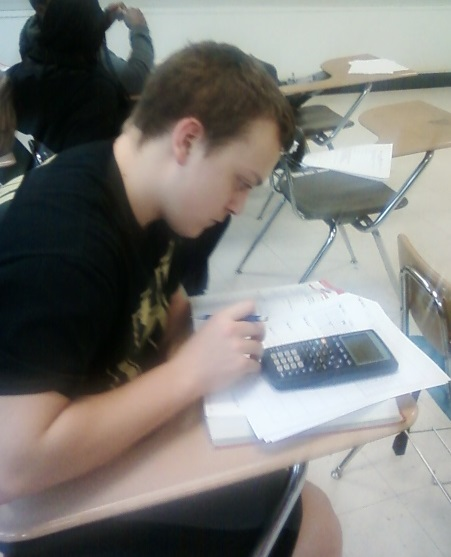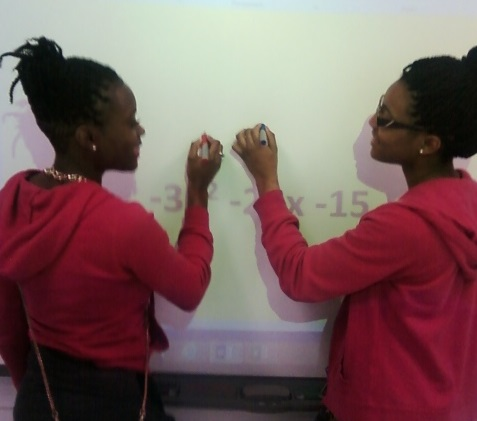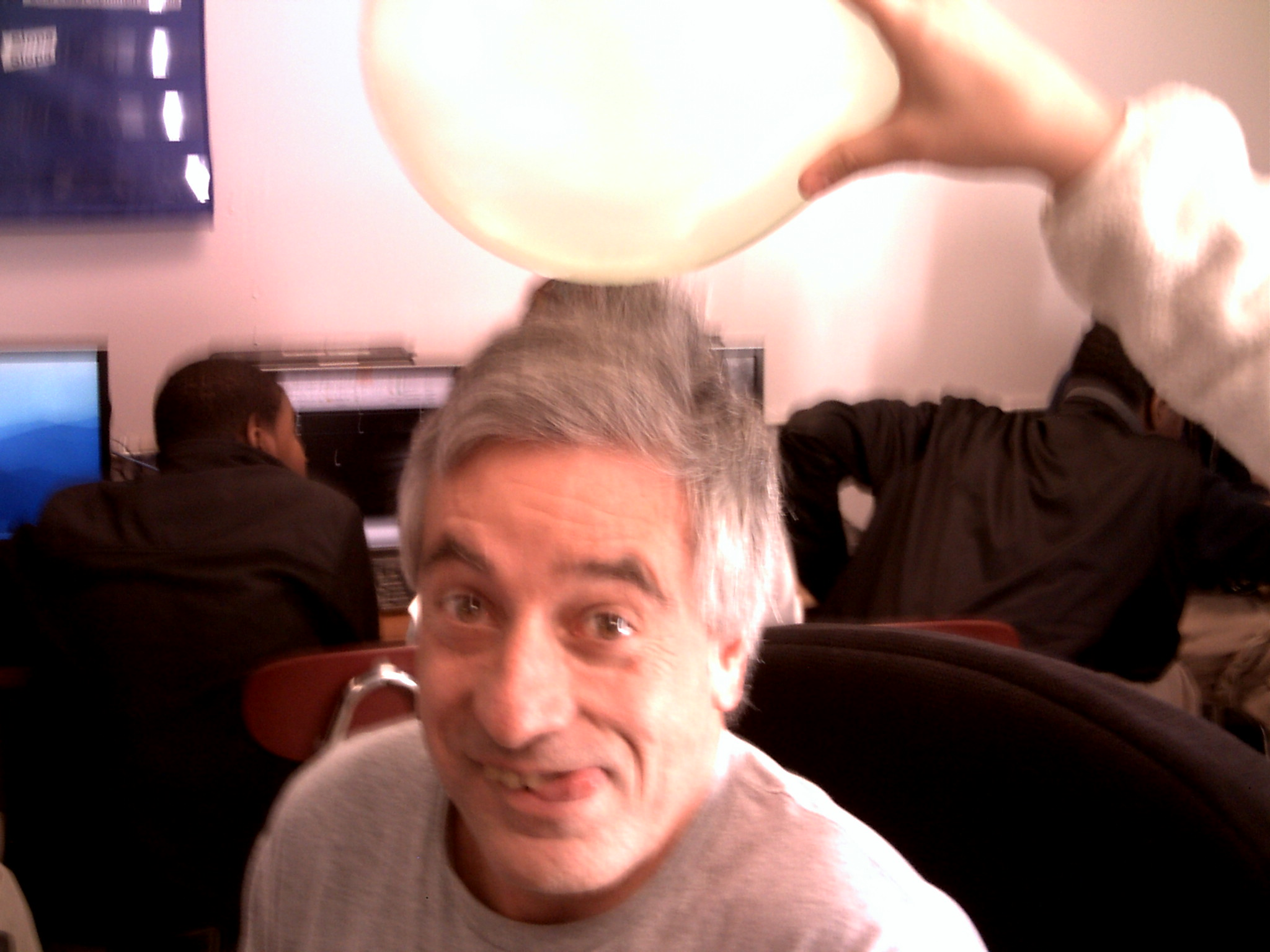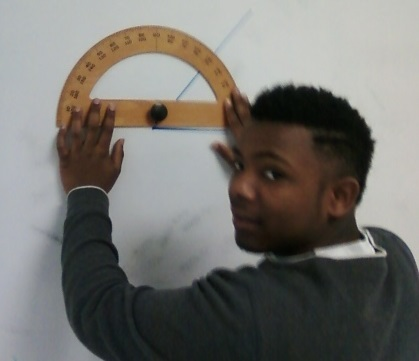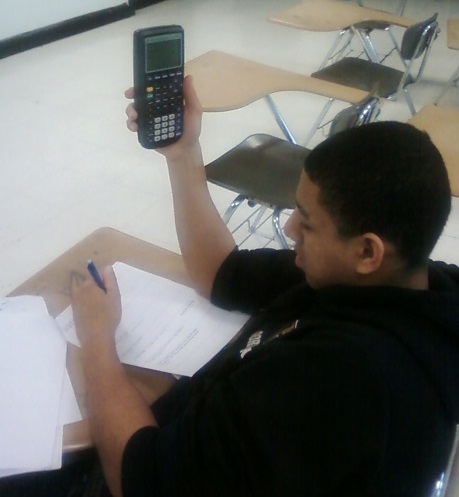Seven sample math problems for the ACT/SAT test given to my students weekly along with my detailed solutions.  Take the quizzes, grade your own work, and try not to cheat yourself!   Note: Sometimes there are different ways to solve a math problem, if you got the correct answer using a logical mathematical process then your solution may not look like my examples; however, you are still accurate and on your journey to a fabulous ACT math score.  rc

7. A  is correct!   From slope intercept form of a line we have y = mx + b  , and we know the slope m is 2.24 and the y-intercept is (0, 16.45) if we change the y-intercept and not the slope our new line must be parallel to the original line. The new y-intercept will be (0 , -3.8).  The line will be lower on our graph.  If you pull the line down the graph keeping the slope positive and parallel then the x-intercept must get larger.  Try it with your pencil on the graph. Place your pencil on the line and move it down keeping it parallel to the line.  If the slope had been negative the opposite would have been true.   However, problem seven is a great example of why you need to read and then throw out the garbage answers.    B can’t be correct as we are decreasing not increasing the y-intercept.  C  and  D can’t be correct because we are not changing the slope at all.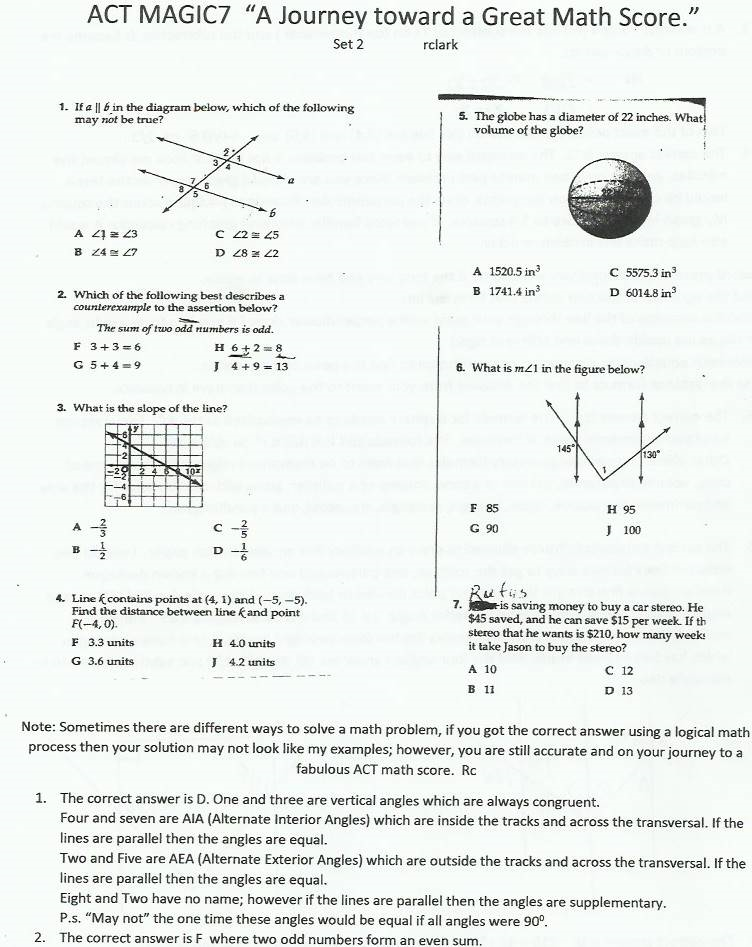2.

Which of the graphs is the solution set of            -3x ≤ 12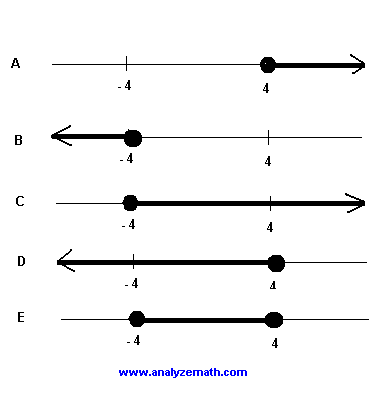5. The lengths in the rectangle below are in inches. What is the shaded area in square inches? a 38             b 39 c 67             d 24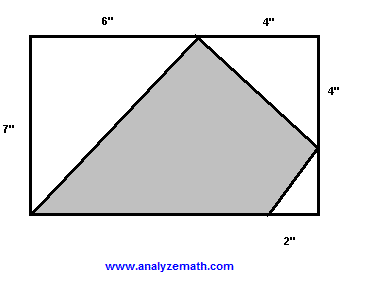1.

A  4                        B  6           C 8                         D   Not enough information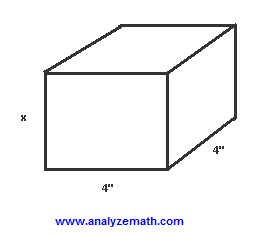Sources for above practice questions:

Varsity Tutors

CrackACT.com Khan Academy ACT Overview